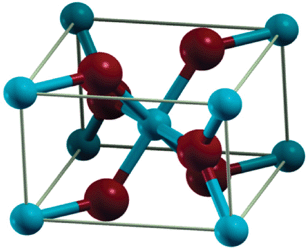# All alkali and alkaline earth metals form ionic hydrides except Be and Mg WHY?? Please reasons, thanks

Jul 24, 2017

Two reasons: (a) ionization energies and (b) electronegativities.

#### Explanation:

(a) Ionization energies

Of all the Group 1 and Group 2 metals, $\text{Be}$ and $\text{Mg}$ have the highest ionization energies (899.5 kJ/mol and 737.7 kJ/mol, respectively).

Thus, it is more difficult to convert them into cations.

(b) Electronegativities

Of all the Group 1 and Group 2 metals, $\text{Be}$ and $\text{Mg}$ have the highest electronegativities (1.57 and 1.31, respectively).

Thus, their electronegativities are the closest to that of $\text{H}$ (2.20).

They are therefore the most likely candidates to have covalent $\text{M-H}$ bonds.

Calculations from electronegativity differences predict a $\text{Be-H}$ bond to have
only 9 % ionic character and an $\text{Mg-H}$ bond to have 18 % ionic character.

The structure of ${\text{BeH}}_{2}$Beryllium hydride forms polymeric chains in which the $\text{H}$ atoms
form 3-centred 2-electron bonds to the next beryllium atom in the chain.

Here's a model of a chain segment.(From www.chemtube3d.com)

The structure of ${\text{MgH}}_{2}$

${\text{MgH}}_{2}$ has a crystal structure in which the $\text{Mg}$ atoms (blue) form a distorted body-centred cubic unit cell.The $\text{Mg}$ is almost completely ionized ($q = \text{+1.95e}$).

However, the $\text{H}$ atoms do not have a corresponding charge of $\text{-1e}$.

Instead there is a significant amount of charge density between $\text{Mg}$ and $\text{H}$, indicating a somewhat covalent $\text{Mg-H}$ bond.